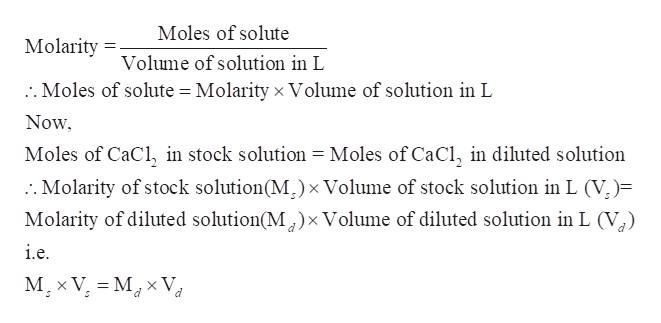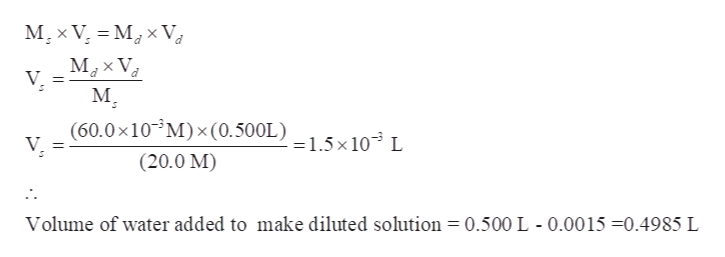You need to make 0.500 L of a 60.0 mM CaCl2 solution from a 20.0 M CaCl2 stock solution. Determine how much stock solution and water you will need to make the dilute solution. Show your calculations.

Question
1. You need to make 0.500 L of a 60.0 mM CaCl2 solution from a 20.0 M CaCl2 stock solution. Determine how much stock solution and water you will need to make the dilute solution. Show your calculations.
Step 1

We have to calculate the volume of stock solution and water required to make 0.500 L of a 60.0 mM CaCl2 solution from a 20.0 M CaCl2 stock solution.

Step 2

On dilution, the amount of solute in the solution does not change. Therefore, Moles of CaCl2 in stock solution would be equal to the moles of CaCl2 in the diluted solution.help_outlineImage TranscriptioncloseMoles of solute Molarity Volume of solution in L :. Moles of solute = Molarity xVolume of solution in L Now Moles of CaCl, in stock solution = Moles of CaCl, in diluted solution :. Molarity of stock solution(M) x Volume of stock solution in L (V)= Molarity of diluted solution(M^) x Volume of diluted solution in L (V i.e M x V M V fullscreen
Step 3

Now, calculate the volume of stock solution using

Ms = 20.0 M

Md = 60.0 mM = 60.0&...help_outlineImage TranscriptioncloseM,x V M x V М,xV, V м, (60.0x10 M) x (0.500L) 1.5x103 L V (20.0 M) Volume of water added to make diluted solution = 0.500 L - 0.0015 -0.4985 L fullscreen

Want to see the full answer?

See Solution

Want to see this answer and more?

Our solutions are written by experts, many with advanced degrees, and available 24/7

See Solution
Tagged in

General Chemistry